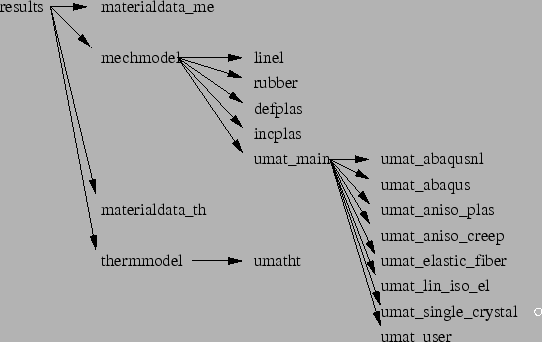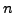Next: Determining the basic characteristics Up: Aerodynamic and hydraulic networks Previous: Aerodynamic and hydraulic networks   Contents

### The variables and the equations

In Sections 6.9.16 and 6.9.17 the governing equations for aerodynamic and hydraulic networks were derived. It was shown that the basic variables for aerodynamic networks are the total temperature, the total pressure and the mass flow. In addition, one geometric parameter may be defined per element as additional unknown. This option has to be coded in the program in order to be active. Right now, this option only exists for the gate valve. All other variables can be calculated based on these three quantities. This is actually not a unique choice but seems to be best suited for our purposes. For hydraulic networks these reduce to the pressure, temperature and mass flow. This is completely different from the structural unknowns, which are taken to be the temperature and the displacements. Therefore, the degrees of freedom 0 to 3 which are used for structural calculations are redefined for networks according to Table (21)

 DOF corner node midside node 0 total temperature - 1 - mass flow 2 total pressure - 3 static temperature geometry

A distinction is being made between corner nodes and midside nodes of fluid elements. Remember that network elements consist of two corner nodes and one middle node (Section 6.2.37). The mass flow is not necessarily uniquely determined at the corner nodes, since more than two branches can come together. Therefore, it is logical to define the mass flow as unknown in the middle of a network element. The same applies to the geometric parameter, if applicable. Similarly, the total temperature or total pressure may not be known within the element, since the exact location of discontinuities (such as enlargements or orifices) is not necessarily known. Consequently, it is advantageous to define the total temperature and total pressure as unknowns in the corner nodes. The static temperature is not a basic variable. Once the total temperature, mass flow and total pressure are known, the static temperature can be calculated. It is a derived quantity.

Similar to field nactdof for structural applications a field nactdog is introduced for network applications. It can be viewed as a matrix with 4 rows and as many columns as there are nodes in the model (including structural nodes; this is done to avoid additional pointing work between the local gas node number and the global node number). It indicates whether a specific degree of freedom in a gas node is active: if the entry is nonzero (actually positive; contrary to nactdof nactdof does not take negative values) it is active, else it is inactive (which means that the value is known or not applicable because the node is a structural node). The degrees of freedom correspond to the first three rows of Table 21 and are repeated in Table 22 for clarity. Here too, only the first three rows are relevant.

 DOF corner node midside node 0 total temperature - 1 - mass flow 2 total pressure - 3 - geometry

Consequently, if nactdog(2,328) is nonzero, it means that the total pressure in node 328 is an unknown in the system. Actually, the nonzero value represents the number of the degree of freedom attached to the total pressure in node 328. The number of the degree of freedom corresponds with the column number in the resulting set of equations. What nactdog is for the degrees of freedom is nacteq for the equations. It is a field of the same size of nactdog but now a nonzero entry indicates that a specific conservation equation applies to the node, cf. Table 23.

 DOF corner node midside node 0 energy - 1 mass - 2 - momentum 3 if0: independent node of isothermal - element the node belongs to;

If nacteq(1,8002) is nonzero, it means that the conservation of mass equation has to be formulated for node 8002. The nonzero value is actually the row number of this equation in the set of equations. If the value is zero, the equation does not apply, e.g. because the mass flow in all adjacent elements is known. The last row in field nacteq (at least for corner nodes) is used to account for isothermal conditions. These only apply to gas pipes of type GAS PIPE ISOTHERMAL and exit restrictors preceded by an isothermal gas pipe element. An isothermal element introduces an extra equation specifying that the static temperature in the two corner nodes of the pipe is equal. This can be transformed into a nonlinear equation in which the total temperature in one node (the dependent node) is written as a function of the total temperature in the other node and the other variables (total pressure in the nodes, mass flow). To account for this extra equation, the conservation of energy is not expressed for the dependent node (indeed, one can argue that, in order for the static temperatures to be equal an unknown amount of heat has to be introduced in the dependent node. So if nacteq(3,8002)=is nonzero it means that node 8002 is the dependent node in an isothermal relation linking the static nodal temperature to the one of node.

Field ineighe(i),i=1,...,ntg is used to determine the static temperature in an end node. If it is zero, node i is a mid-node. If it is equal to -1, the node is a chamber, for which the static temperature equals the total temperature. If it is positive, its value is the element number of a gas pipe element or restrictor element, but not equal to a restrictor wall orifice, for which the static temperature is different from the total temperature. The mass flow of the referred element is used to calculate the static temperature from the total temperature.Next: Determining the basic characteristics Up: Aerodynamic and hydraulic networks Previous: Aerodynamic and hydraulic networks   Contents
guido dhondt 2018-12-15